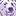# New to Qlik Sense

If you’re new to Qlik Sense, start with this Discussion Board and get up-to-speed quickly.

Announcements
Join us for a live Q&A! September 21, 10 AM ET - Onboarding Fast in Qlik Sense SaaS! REGISTER
cancel
Showing results for
Did you mean:Contributor III

## Variable change field in a table - Qliksense

Hi everybody,

I am trying to fix a problem I am having on QlikSense. So what I am trying to do is to change a value of a variable based on the value selected in another. And it all works but the last variable I have is inserted as a dimension in a table. And when I change the value in the first variable, in the input box it shows me the value changed but it doesn't change the value in the table. In fact it changes the value only if I select another value in the last variable and then I re-select the value changed.

So let's suppose I have two variables:

- v1 which can be equal to Budget, Stat, Comm

- v2 which can be equal to 'Nation', 'Month', and if(v1=Budget, 'Budget Agent', 'Dest Agent')

(The values put on v2 are equal to the field I want to analyze on the table)

So let's suppose I have selected v1=Stat and v2=Dest Agent, and the I select budget in v1. So it changes the value shows on v2 but it doesn't change the dimension in the table.

Is there a way to achieve what I am trying to do.

Thanks

1 Solution

Accepted SolutionsCreator II

Thanks for the QVF file, i understood the issue better with the sample file. The issue here is the condition used, when you select the first variable (Document type) you want to dynamically change the value for dimension analysis (Nazione / BP). The problem here is that when you have already made the selection and you want to update the selection the variable has to refresh and this was not happening.

To rectify this, i made few changes. instead of assigning string values to the variable i used numbers

Var1 is the variable used for Document type and the labels and values are (Invoiced = 1, Orders=2),

Var2 is the variable used for analysis dimension and the labels and values are (Agente BP =1, Mese = 2 and if(\$(var1)=1,'BP','Nazione'), 3)

additionally, i have created a new variable called "New_Dim" and its value is

pick(Match(var2,3)+1
,Pick(Match(var2,1,2),chr(91)&'Agente BP'&chr(93),'Mese')
,Pick(var1,'BP','Nazione'))

and then refer this variable in Dimension of the table as \$(New_Dim) and this should allow you display the values dynamically.

also, i have attached your QVF file for reference.

6 RepliesCreator II

if you are using a column name as Dest Agent, you need to pass this column value as "Dest Agent" or [Dest Agent], because of this qlik is unable to read the column name. either you make changes to the variable or rename the column as "Dest_Agent" and use this as reference.Contributor III
Author

I tried to put as variable value directly the field name without putting it like this 'Nation', but the table doesn't recognize the dimension either if I put my variable as a dimension in this way \$(v2) or directly as v2.

I attach the photos of what happens. As you can see when I change my first variable from 'Invoiced' to ''Orders' the second one changes it's value from 'BP' to 'Nazione' but the table dimension which is equal to \$(v2) doesn't change. In fact it changes correctly to 'Nazione' only if I select for example 'Agente BP' and then I reselect 'Nazione'

The expression I use to make the variable change based on the value of my first variable is:

=if(v1='Invoiced','Nazione','BP')

Thank you so much

ZenoCreator II

in your variable expression, do not include =, it should be if(v1='Invoiced','Nazione','BP') and you can parse the variable in expression as \$(v2)Contributor III
Author

I tried your solution and it changes correctly the value showed in my variable input (for v2) but it doesn't change the value in the table (until I select another value and then reselect the value). It is like Qliksense doesn't recognize the change happened until I reselect it.

I attach here a simulation of my app.

I hope that this can help.

Thanks,

ZenoCreator II

Thanks for the QVF file, i understood the issue better with the sample file. The issue here is the condition used, when you select the first variable (Document type) you want to dynamically change the value for dimension analysis (Nazione / BP). The problem here is that when you have already made the selection and you want to update the selection the variable has to refresh and this was not happening.

To rectify this, i made few changes. instead of assigning string values to the variable i used numbers

Var1 is the variable used for Document type and the labels and values are (Invoiced = 1, Orders=2),

Var2 is the variable used for analysis dimension and the labels and values are (Agente BP =1, Mese = 2 and if(\$(var1)=1,'BP','Nazione'), 3)

additionally, i have created a new variable called "New_Dim" and its value is

pick(Match(var2,3)+1
,Pick(Match(var2,1,2),chr(91)&'Agente BP'&chr(93),'Mese')
,Pick(var1,'BP','Nazione'))

and then refer this variable in Dimension of the table as \$(New_Dim) and this should allow you display the values dynamically.

also, i have attached your QVF file for reference.Contributor III
Author

Thanks! This was great helpTags
Community Browser